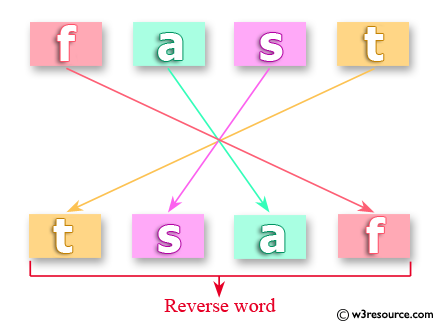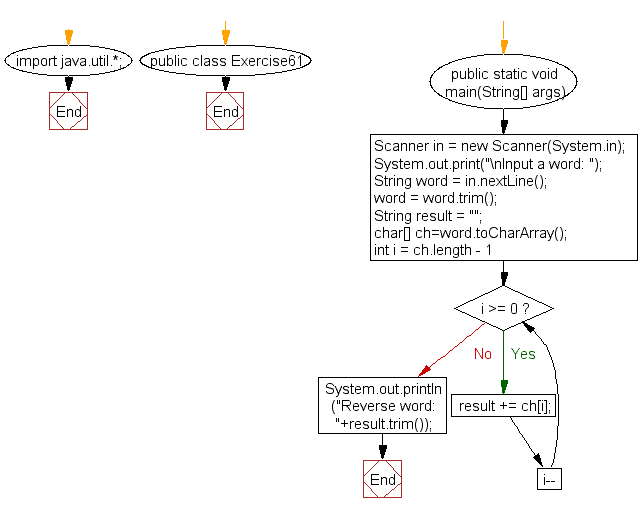﻿ Java exercises: Reverse a word - w3resource# Java Exercises: Reverse a word

## Java Basic: Exercise-61 with Solution

Write a Java program to reverse a word.

Pictorial Presentation: Reverse a wordSample Solution:

Java Code:

``````import java.util.*;
public class Exercise61 {
public static void main(String[] args){
Scanner in = new Scanner(System.in);
System.out.print("\nInput a word: ");
String word = in.nextLine();
word = word.trim();
String result = "";
char[] ch=word.toCharArray();
for (int i = ch.length - 1; i >= 0; i--) {
result += ch[i];
}
System.out.println("Reverse word: "+result.trim());
}
}
```
```

Sample Output:

```Input a word: dsaf
Reverse word: fasd
```

Flowchart:Java Code Editor:

What is the difficulty level of this exercise?

Test your Programming skills with w3resource's quiz.

﻿

## Java: Tips of the Day

countOccurrences

Counts the occurrences of a value in an array.

Use Arrays.stream().filter().count() to count total number of values that equals the specified value.

```public static long countOccurrences(int[] numbers, int value) {
return Arrays.stream(numbers)
.filter(number -> number == value)
.count();
}
```

Ref: https://bit.ly/3kCAgLb